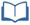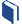# X…Y…Zzzzzzz

Estimated Time: 90 minutes

###Lesson Objectives:

• The students will determine population percentages by calculating areas under the normal curve.
• The students will use tools strategically to solve real-world applications that involve estimating areas under the normal curve.

###Resources for this lesson:

Before you begin, download your Algebra II Journalfor X…Y…Zzzzzzz. You will be completing activities in the journal throughout this lesson.

Visit this section on each page to access information about key terms and other resources to help facilitate the lesson.

> Glossary> Calculator Resources> Teacher Resources: Instructional NotesKey Common Core State Standards:

S.ID.4: Use the mean and standard deviation of a data set to fit it to a normal distribution and to estimate population percentages. Recognize that there are data sets for which such a procedure is not appropriate. Use calculators, spreadsheets, and tables to estimate areas under the normal curve.

Supporting Common Core State Standards:

N/A

Standards for Mathematical Practices:

1. Make sense of problems and persevere in solving them.
2. Reason abstractly and quantitatively.
4. Model with mathematics.
5. Use appropriate tools strategically.
6. Attend to precision.
7. Look for and make use of structure.
8. Look for and express regularity in repeated reasoning.

Next >